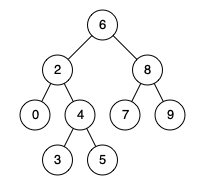Lowest Common Ancestor of a Binary Search Tree

Given a binary search tree (BST), find the lowest common ancestor (LCA) of two given nodes in the BST.

According to the definition of LCA on Wikipedia: “The lowest common ancestor is defined between two nodes p and q as the lowest node in T that has both p and q as descendants (where we allow a node to be a descendant of itself).”

Given binary search tree: root = [6,2,8,0,4,7,9,null,null,3,5]Example 1:

Input:
root = [6,2,8,0,4,7,9,null,null,3,5], p = 2, q = 8

Output:
6

Explanation:
The LCA of nodes 2 and 8 is 6.

Example 2:

Input:
root = [6,2,8,0,4,7,9,null,null,3,5], p = 2, q = 4

Output:
2

Explanation:
The LCA of nodes 2 and 4 is 2
, since a node can be a descendant of itself according to the LCA definition.

Note:

• All of the nodes' values will be unique.
• p and q are different and both values will exist in the BST.

Solution & Analysis

Using attribution of a BST

/**
* Definition for a binary tree node.
* public class TreeNode {
*     int val;
*     TreeNode left;
*     TreeNode right;
*     TreeNode(int x) { val = x; }
* }
*/
class Solution {
public TreeNode lowestCommonAncestor(TreeNode root, TreeNode p, TreeNode q) {

// Value of current node or parent node.
int parentVal = root.val;

// Value of p
int pVal = p.val;

// Value of q;
int qVal = q.val;

if (pVal > parentVal && qVal > parentVal) {
// If both p and q are greater than parent
return lowestCommonAncestor(root.right, p, q);
} else if (pVal < parentVal && qVal < parentVal) {
// If both p and q are lesser than parent
return lowestCommonAncestor(root.left, p, q);
} else {
// We have found the split point, i.e. the LCA node.
return root;
}
}
}

Iterative

Same code as Lowest Common Ancestor of a Binary Tree

public class Solution {
public TreeNode lowestCommonAncestor(TreeNode root, TreeNode p, TreeNode q) {
if(root == null || root == p || root == q) return root;
TreeNode left = lowestCommonAncestor(root.left, p, q);
TreeNode right = lowestCommonAncestor(root.right, p, q);
if(left != null && right != null) return root;
return left != null ? left : right;
}
}

Reference

https://www.lintcode.com/problem/lowest-common-ancestor-ii/description

https://www.lintcode.com/problem/lowest-common-ancestor-iii/description